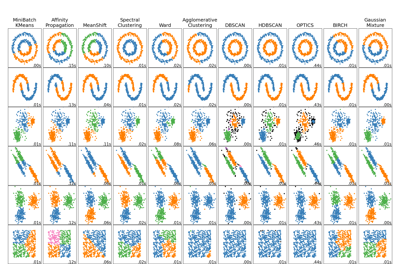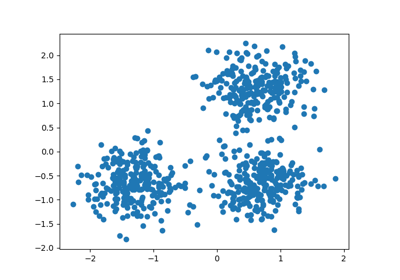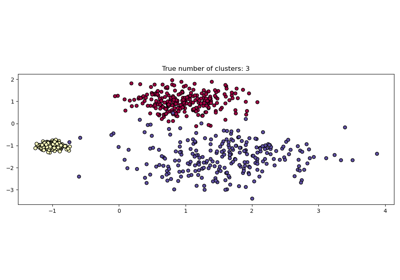# sklearn.cluster.DBSCAN¶

class sklearn.cluster.DBSCAN(eps=0.5, *, min_samples=5, metric='euclidean', metric_params=None, algorithm='auto', leaf_size=30, p=None, n_jobs=None)[source]

Perform DBSCAN clustering from vector array or distance matrix.

DBSCAN - Density-Based Spatial Clustering of Applications with Noise. Finds core samples of high density and expands clusters from them. Good for data which contains clusters of similar density.

The worst case memory complexity of DBSCAN is $$O({n}^2)$$, which can occur when the eps param is large and min_samples is low.

Read more in the User Guide.

Parameters:
epsfloat, default=0.5

The maximum distance between two samples for one to be considered as in the neighborhood of the other. This is not a maximum bound on the distances of points within a cluster. This is the most important DBSCAN parameter to choose appropriately for your data set and distance function.

min_samplesint, default=5

The number of samples (or total weight) in a neighborhood for a point to be considered as a core point. This includes the point itself. If min_samples is set to a higher value, DBSCAN will find denser clusters, whereas if it is set to a lower value, the found clusters will be more sparse.

metricstr, or callable, default=’euclidean’

The metric to use when calculating distance between instances in a feature array. If metric is a string or callable, it must be one of the options allowed by sklearn.metrics.pairwise_distances for its metric parameter. If metric is “precomputed”, X is assumed to be a distance matrix and must be square. X may be a sparse graph, in which case only “nonzero” elements may be considered neighbors for DBSCAN.

New in version 0.17: metric precomputed to accept precomputed sparse matrix.

metric_paramsdict, default=None

Additional keyword arguments for the metric function.

New in version 0.19.

algorithm{‘auto’, ‘ball_tree’, ‘kd_tree’, ‘brute’}, default=’auto’

The algorithm to be used by the NearestNeighbors module to compute pointwise distances and find nearest neighbors. See NearestNeighbors module documentation for details.

leaf_sizeint, default=30

Leaf size passed to BallTree or cKDTree. This can affect the speed of the construction and query, as well as the memory required to store the tree. The optimal value depends on the nature of the problem.

pfloat, default=None

The power of the Minkowski metric to be used to calculate distance between points. If None, then p=2 (equivalent to the Euclidean distance).

n_jobsint, default=None

The number of parallel jobs to run. None means 1 unless in a joblib.parallel_backend context. -1 means using all processors. See Glossary for more details.

Attributes:
core_sample_indices_ndarray of shape (n_core_samples,)

Indices of core samples.

components_ndarray of shape (n_core_samples, n_features)

Copy of each core sample found by training.

labels_ndarray of shape (n_samples)

Cluster labels for each point in the dataset given to fit(). Noisy samples are given the label -1.

n_features_in_int

Number of features seen during fit.

New in version 0.24.

feature_names_in_ndarray of shape (n_features_in_,)

Names of features seen during fit. Defined only when X has feature names that are all strings.

New in version 1.0.

OPTICS

A similar clustering at multiple values of eps. Our implementation is optimized for memory usage.

Notes

For an example, see examples/cluster/plot_dbscan.py.

This implementation bulk-computes all neighborhood queries, which increases the memory complexity to O(n.d) where d is the average number of neighbors, while original DBSCAN had memory complexity O(n). It may attract a higher memory complexity when querying these nearest neighborhoods, depending on the algorithm.

One way to avoid the query complexity is to pre-compute sparse neighborhoods in chunks using NearestNeighbors.radius_neighbors_graph with mode='distance', then using metric='precomputed' here.

Another way to reduce memory and computation time is to remove (near-)duplicate points and use sample_weight instead.

OPTICS provides a similar clustering with lower memory usage.

References

Ester, M., H. P. Kriegel, J. Sander, and X. Xu, “A Density-Based Algorithm for Discovering Clusters in Large Spatial Databases with Noise”. In: Proceedings of the 2nd International Conference on Knowledge Discovery and Data Mining, Portland, OR, AAAI Press, pp. 226-231. 1996

Schubert, E., Sander, J., Ester, M., Kriegel, H. P., & Xu, X. (2017). “DBSCAN revisited, revisited: why and how you should (still) use DBSCAN.” ACM Transactions on Database Systems (TODS), 42(3), 19.

Examples

>>> from sklearn.cluster import DBSCAN
>>> import numpy as np
>>> X = np.array([[1, 2], [2, 2], [2, 3],
...               [8, 7], [8, 8], [25, 80]])
>>> clustering = DBSCAN(eps=3, min_samples=2).fit(X)
>>> clustering.labels_
array([ 0,  0,  0,  1,  1, -1])
>>> clustering
DBSCAN(eps=3, min_samples=2)


Methods

 fit(X[, y, sample_weight]) Perform DBSCAN clustering from features, or distance matrix. fit_predict(X[, y, sample_weight]) Compute clusters from a data or distance matrix and predict labels. Get metadata routing of this object. get_params([deep]) Get parameters for this estimator. set_fit_request(*[, sample_weight]) Request metadata passed to the fit method. set_params(**params) Set the parameters of this estimator.
fit(X, y=None, sample_weight=None)[source]

Perform DBSCAN clustering from features, or distance matrix.

Parameters:
X{array-like, sparse matrix} of shape (n_samples, n_features), or (n_samples, n_samples)

Training instances to cluster, or distances between instances if metric='precomputed'. If a sparse matrix is provided, it will be converted into a sparse csr_matrix.

yIgnored

Not used, present here for API consistency by convention.

sample_weightarray-like of shape (n_samples,), default=None

Weight of each sample, such that a sample with a weight of at least min_samples is by itself a core sample; a sample with a negative weight may inhibit its eps-neighbor from being core. Note that weights are absolute, and default to 1.

Returns:
selfobject

Returns a fitted instance of self.

fit_predict(X, y=None, sample_weight=None)[source]

Compute clusters from a data or distance matrix and predict labels.

Parameters:
X{array-like, sparse matrix} of shape (n_samples, n_features), or (n_samples, n_samples)

Training instances to cluster, or distances between instances if metric='precomputed'. If a sparse matrix is provided, it will be converted into a sparse csr_matrix.

yIgnored

Not used, present here for API consistency by convention.

sample_weightarray-like of shape (n_samples,), default=None

Weight of each sample, such that a sample with a weight of at least min_samples is by itself a core sample; a sample with a negative weight may inhibit its eps-neighbor from being core. Note that weights are absolute, and default to 1.

Returns:
labelsndarray of shape (n_samples,)

Cluster labels. Noisy samples are given the label -1.

Get metadata routing of this object.

Please check User Guide on how the routing mechanism works.

Returns:

A MetadataRequest encapsulating routing information.

get_params(deep=True)[source]

Get parameters for this estimator.

Parameters:
deepbool, default=True

If True, will return the parameters for this estimator and contained subobjects that are estimators.

Returns:
paramsdict

Parameter names mapped to their values.

set_fit_request(*, sample_weight: Union[bool, None, str] = '$UNCHANGED$') [source]

Request metadata passed to the fit method.

Note that this method is only relevant if enable_metadata_routing=True (see sklearn.set_config). Please see User Guide on how the routing mechanism works.

The options for each parameter are:

• True: metadata is requested, and passed to fit if provided. The request is ignored if metadata is not provided.

• False: metadata is not requested and the meta-estimator will not pass it to fit.

• None: metadata is not requested, and the meta-estimator will raise an error if the user provides it.

• str: metadata should be passed to the meta-estimator with this given alias instead of the original name.

The default (sklearn.utils.metadata_routing.UNCHANGED) retains the existing request. This allows you to change the request for some parameters and not others.

New in version 1.3.

Note

This method is only relevant if this estimator is used as a sub-estimator of a meta-estimator, e.g. used inside a Pipeline. Otherwise it has no effect.

Parameters:
sample_weightstr, True, False, or None, default=sklearn.utils.metadata_routing.UNCHANGED

Metadata routing for sample_weight parameter in fit.

Returns:
selfobject

The updated object.

set_params(**params)[source]

Set the parameters of this estimator.

The method works on simple estimators as well as on nested objects (such as Pipeline). The latter have parameters of the form <component>__<parameter> so that it’s possible to update each component of a nested object.

Parameters:
**paramsdict

Estimator parameters.

Returns:
selfestimator instance

Estimator instance.

## Examples using sklearn.cluster.DBSCAN¶Comparing different clustering algorithms on toy datasets

Comparing different clustering algorithms on toy datasetsDemo of DBSCAN clustering algorithm

Demo of DBSCAN clustering algorithmDemo of HDBSCAN clustering algorithm

Demo of HDBSCAN clustering algorithm